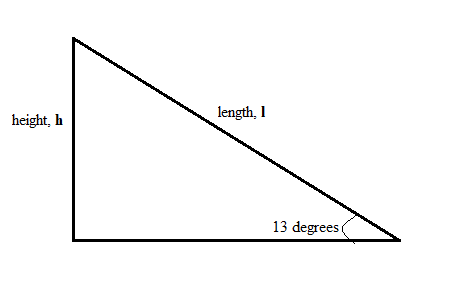# A skier is gliding along at 5.0 m/s on horizontal, frictionless snow. He suddenly starts down a...

## Question:

A skier is gliding along at 5.0 m/s on horizontal, frictionless snow. He suddenly starts down a 13 degrees incline. His speed at the bottom is 12 m/s. What is the length of the incline? How long does it take him to reach the bottom?

## Energy Conservation:

The total energy of an isolated system remains constant at all times. This means any changes in the energy of the system will cancel each other off and the total energy is maintained constant.

In our case, we have a skier of mass m whose kinetic energy changed from

{eq}0.5 \ m \ \times \ (5.0 \ m/s)^2 \ = \ 12.5 \ \times \ m {/eq}

to

{eq}0.5 \ m \ \times \ (12 \ m/s)^2 \ = \ 72 \ \times \ m {/eq}

both in Joules. The gain in kinetic energy is

{eq}\Delta K.E \ = \ 59.5 \ \times \ m {/eq}

From conservation of energy, this is the potential energy lost by the skier from the top to the bottom. Let the height he falls be h. Therefore, the change in potential energy is

{eq}\Delta U \ = \ m \ \times \ 9.81 \ m/s^2 \ \times \ h {/eq}

Equating the two expressions, we get

{eq}\begin{align*} \Delta U \ &= \ \Delta K.E\\ \\ m \ \times \ 9.81 \ \times \ h \ &= \ 59.5 \ \times \ m\\ \\ \therefore \ h \ &= \ \dfrac{59.5}{9.81}\\ \\ &= \ 6.065239551 \ m \end{align*} {/eq}

The diagram below shows the height h and the length of the incline l.Therefore, the length of the incline is

{eq}l \ = \ \dfrac{h}{\sin 13^{\circ}} \ = \ \dfrac{6.065239551 \ m}{\sin 13^{\circ}} \ = \ 26.96248555 \ m \ = \ \mathbf{27.0 \ m} {/eq}

correct to three significant figures.

The time taken by the skier to reach the bottom is given by dividing the length of the incline by the average speed. The average speed is

{eq}v_{avg} \ = \ 0.5 \ \times \ (5.0 \ + \ 12) \ m/s \ = \ 8.5 \ m/s {/eq}

Therefore, the time is

{eq}t \ = \ \dfrac{l}{v_{avg}} \ = \ \dfrac{26.96248555 \ m}{8.5 \ m/s} \ = \ \mathbf{3.17 \ s} {/eq}

correct to three significant figures.